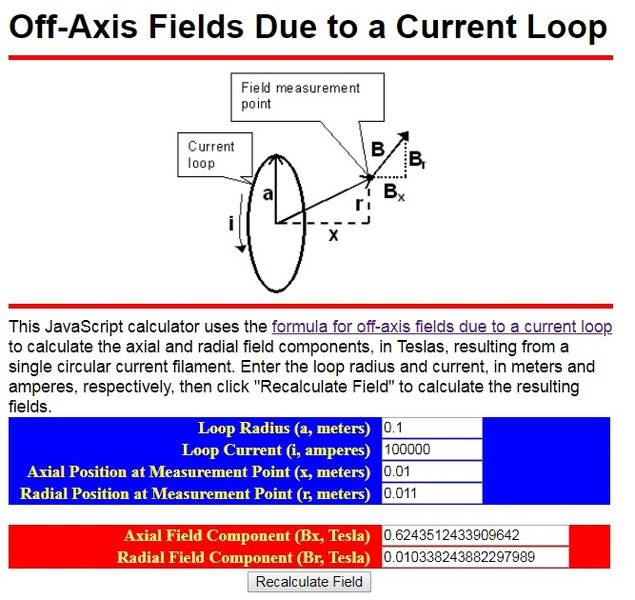# What is the magnetic field off-axis in the y direction?

C++ software using elliptics integral at: https://tiggerntatie.github.io/emagnet/offaxis/elliptic_c.htm
And the calculator here: https://tiggerntatie.github.io/emagnet/offaxis/iloopcalculator.htm
I placed some values and obtained Br smaller than Bx, so I do not know how to calculate By (perpendicular to the axis) unless it is imaginary :) :#### Attachments

Ibix
What formula did you try to implement?

Have you tried calculating manually (elliptic integral tables are available for download) to see if the implementation is correct?

If your diagram is correctly labelled, ##B_r^2=B_y^2+B_z^2##, so I don't understand why you regard ##B_r<B_x## as a problem anyway.

•Javier Lopez
Ok, I understand, you say that Br is not the diagonal but the vertical axel, then I can test using on axis formula, also the elliptics.

Last edited:
Ibix
Ok, I understand, you say that Br is not the diagonal but the vertical axle, then I can test using on axis formula, also the elliptics.
##B_r## is radial, which happens to be vertical in the diagram, yes.

Thank you.
I tested it using axial formula and was OK, but I found an error in the c++ program: if r<0 then the loop not finalizes, so I wote an "if" at the begin that if r<0 r=-r and a flag is activated to make Bz=-Bz at the end

I tested also making a long radii loop and measuring close to the wire (that is the worse case) to check again field close to a long wire.
I stated at the result an incredible accuracy:
At 1mm of a long wire it is expected to have 2.2 Teslas